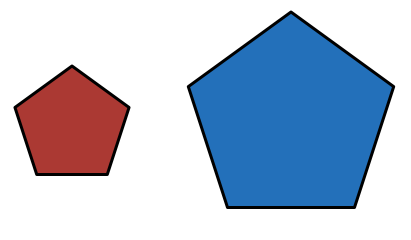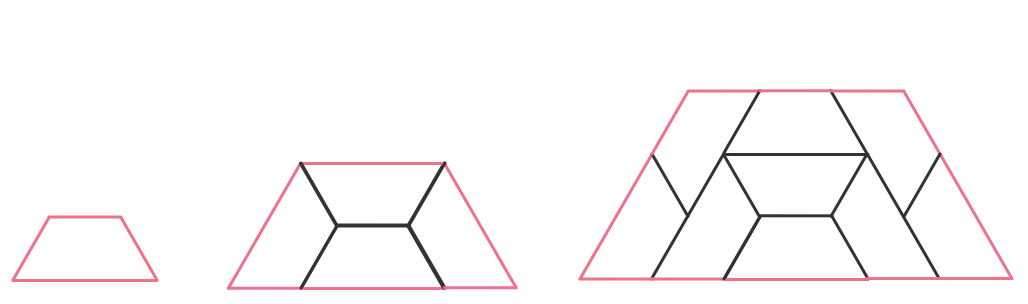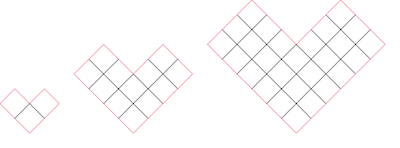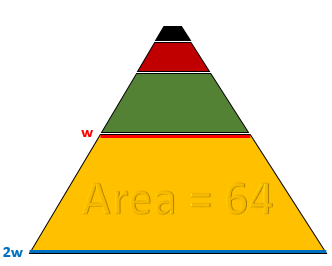Geometry

# Similar Polygons - Problem SolvingTwo similar polygons have areas in the ratio of $16 : 25$. What is the ratio of their perimeters?In this sequence of growing trapezoids, each trapezoid is constructed out of some number of smaller, similar "unit trapezoids" where the three shorter sides are each equal to 1 unit in length and the longer side is equal to 2 units in length. The first figure in the sequence is a single unit trapezoid, the next figure contains 4, the next is made of 9...

What is the perimeter of a trapezoid made out of 100 unit-trapezoids?In this sequence of similar, growing "V" figures, each V is constructed out of some number of identical small, square tiles.

What is the perimeter of the next, similar V-figure in this sequence, which would be made out of 48 square tiles?

2 regular decagons (10-sided) have sides lengths 16 cm and 30 cm. A third regular decagon has area that is equal to the sum of areas of the other 2 decagons. What is the side length (in cm) of the third regular decagon?If the yellow, green, red, and black trapezoids in this figure are all similar, and if the yellow trapezoid has area 64, what is the area of the entire figure (yellow + green + red + black)?

Hint: Can you use the ratio of scale between the yellow and green trapezoids to calculate the area of the green trapezoid quickly?...

×